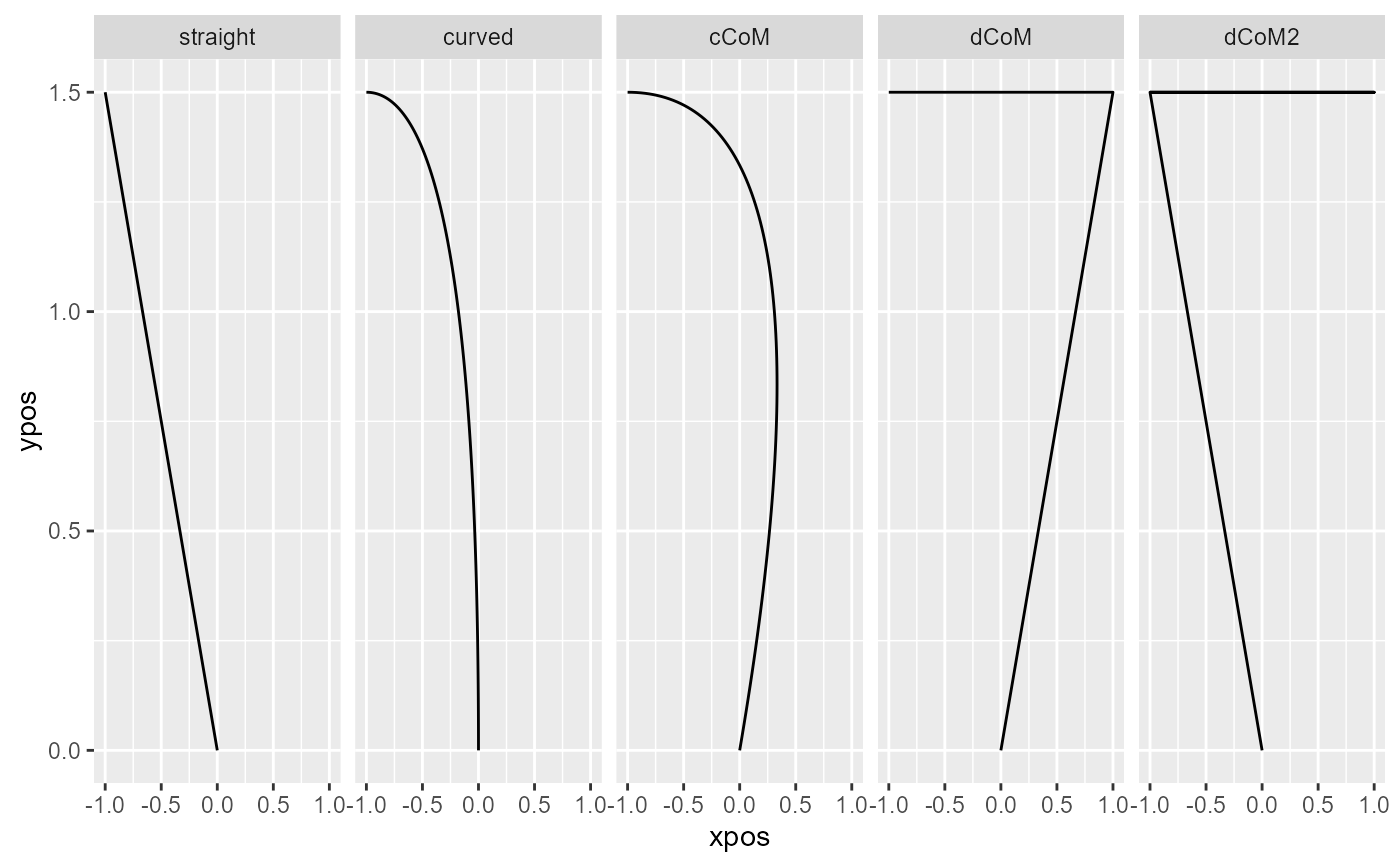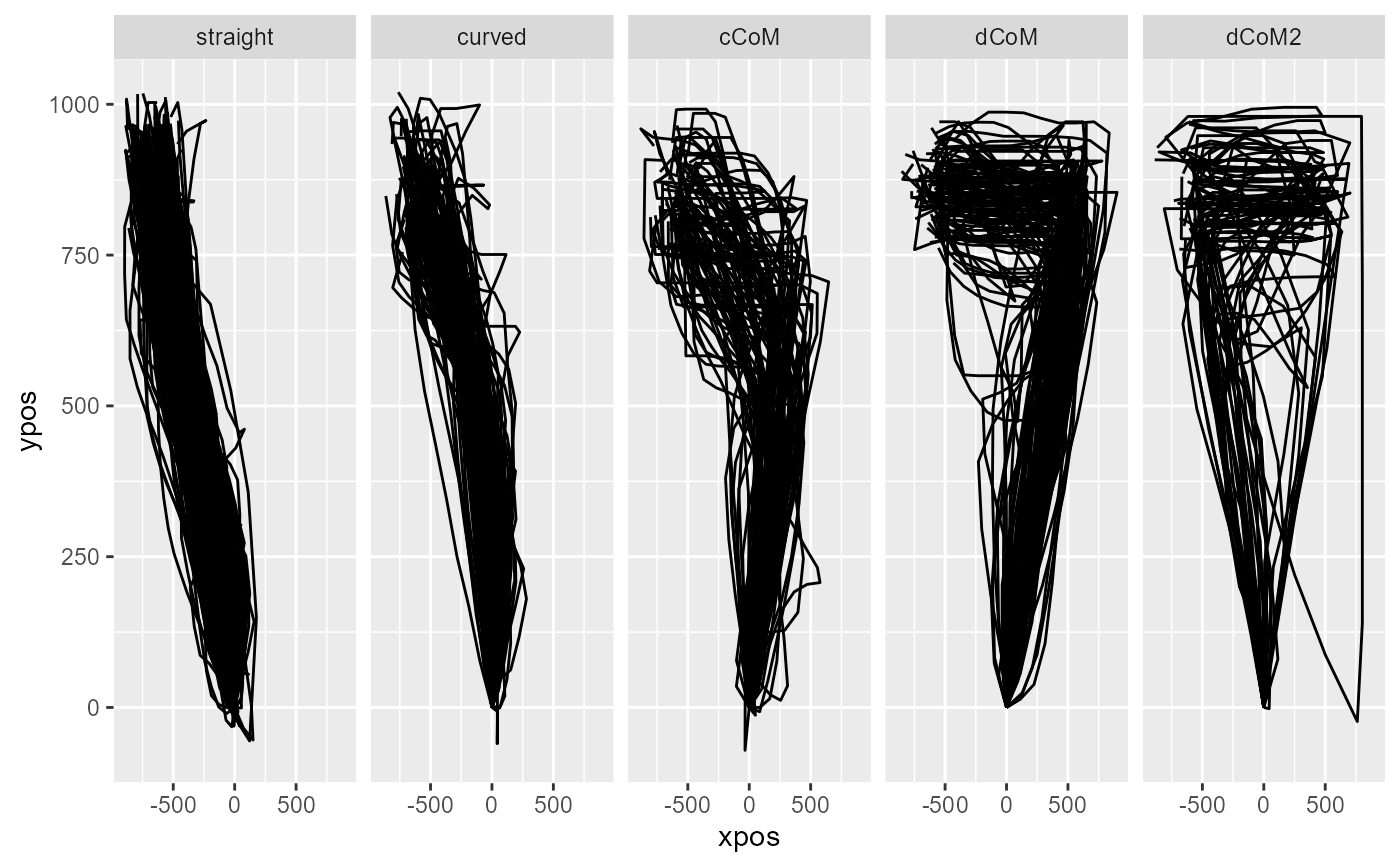mt_map maps trajectories onto a predefined set of prototype trajectories. It first computes distances between the trajectories and each of the supplied trajectory types and then assigns each trajectory to the prototype that produced the smallest distance.

mt_map(
data,
use = "sp_trajectories",
save_as = "prototyping",
dimensions = c("xpos", "ypos"),
prototypes = mousetrap::mt_prototypes,
weights = rep(1, length(dimensions)),
pointwise = TRUE,
na_rm = FALSE,
minkowski_p = 2,
use2 = "data",
grouping_variables = NULL
)

## Arguments

data a mousetrap data object created using one of the mt_import functions (see mt_example for details). Alternatively, a trajectory array can be provided directly (in this case use will be ignored). a character string specifying which trajectory data should be used. a character string specifying where the resulting data should be stored. a character vector specifying which trajectory variables should be used. Can be of length 2 or 3 for two-dimensional or three-dimensional trajectories respectively. a trajectory array containing the prototypes the trajectories are mapped to. As a starting point, the trajectories stored in mt_prototypes can be used. See Details and Examples for selecting prototypes and creating new ones. numeric vector specifying the relative importance of the variables specified in dimensions. Defaults to a vector of 1s implying equal importance. Technically, each variable is rescaled so that the standard deviation matches the corresponding value in weights. To use the original variables, set weights = NULL. boolean specifying the way dissimilarity between the trajectories is measured (see Details). If TRUE (the default), mt_distmat measures the average dissimilarity and then sums the results. If FALSE, mt_distmat measures dissimilarity once (by treating the various points as independent dimensions). logical specifying whether trajectory points containing NAs should be removed. Removal is done column-wise. That is, if any trajectory has a missing value at, e.g., the 10th recorded position, the 10th position is removed for all trajectories. This is necessary to compute distance between trajectories. an integer specifying the distance metric. minkowski_p = 1 computes the city-block distance, minkowski_p = 2 (the default) computes the Euclidian distance, minkowski_p = 3 the cubic distance, etc. an optional character string specifying where the data that contain the variables used for grouping can be found (in case grouping_variables are specified). Defaults to "data" as data[["data"]] usually contains all non mouse-tracking trial data. a character string (or vector) specifying one or more variables in use2. If specified, prototypes will be rescaled separately to match the coordinate system of the trajectories for each level of the variable(s). If unspecified (the default), the prototypes are rescaled in the same way across all trajectories.

## Value

A mousetrap data object (see mt_example) with an additional data.frame (by default called prototyping) that contains the best fitting prototype for each trajectory (the number of the prototype is specified under prototype, the label of the prototype under prototype_label) and the distance of the trajectory to the best fitting prototype (min_dist). If a trajectory array was provided directly as data, only the data.frame containing the results will be returned.

## Details

Mouse trajectories often occur in distinct, qualitative types (see Wulff et al., in press; Wulff et al., 2018). Common trajectory types are linear trajectories, mildly and strongly curved trajctories, and single and multiple change-of-mind trials. mt_map allows to map trajectories to a predefined set of trajectory types.

First, mt_map adjusts prototypes to match the coordinate system of the trajectories specified by use. Next, mt_map computes the distances between each trajectory and each of the supplied prototypes (see mt_distmat) and then assigns each trajectory to the closest prototype (i.e., the prototype that produced the smallest distance).

Mapping trajectories to prototypes requires that the endpoints of all trajectories (and added prototypes) share the same direction, i.e., that all trajectories end in the top-left corner of the coordinate system (mt_remap_symmetric or mt_align can be used to achieve this). Furthermore, it is recommended to use spatialized trajectories (see mt_spatialize; Wulff et al., in press; Haslbeck et al., 2018).

Wulff, D. U., Haslbeck, J. M. B., Kieslich, P. J., Henninger, F., & Schulte-Mecklenbeck, M. (2019). Mouse-tracking: Detecting types in movement trajectories. In M. Schulte-Mecklenbeck, A. Kühberger, & J. G. Johnson (Eds.), A Handbook of Process Tracing Methods (pp. 131-145). New York, NY: Routledge.

Wulff, D. U., Haslbeck, J. M. B., & Schulte-Mecklenbeck, M. (2018). Measuring the (dis-)continuous mind: What movement trajectories reveal about cognition. Manuscript in preparation.

Haslbeck, J. M. B., Wulff, D. U., Kieslich, P. J., Henninger, F., & Schulte-Mecklenbeck, M. (2018). Advanced mouse- and hand-tracking analysis: Detecting and visualizing clusters in movement trajectories. Manuscript in preparation.

## Examples

# Spatialize trajectories
KH2017 <- mt_spatialize(KH2017)

# Map trajectories onto standard prototype set
KH2017 <- mt_map(KH2017,
use="sp_trajectories")

# Plot prototypes
mt_plot(mt_prototypes,facet_col="mt_id") +
ggplot2::facet_grid(.~factor(mt_id,levels=unique(mt_id)))# Plot trajectories per assigned prototype
mt_plot(KH2017,use="sp_trajectories",
use2="prototyping",facet_col="prototype_label")# Map trajectories onto reduced prototype set
KH2017 <- mt_map(KH2017,
use="sp_trajectories",
prototypes=mt_prototypes[c("straight","curved","cCoM"),,],
save_as="prototyping_red")

# Map trajectories onto extended prototype set

# Add additional prototypes
mt_prototypes_ext <- mt_add_trajectory(mt_prototypes,
xpos = c(0,1,-1,1,-1), ypos = c(0,1.5,1.5,1.5,1.5), id = "dCoM3"
)
mt_prototypes_ext <- mt_add_trajectory(mt_prototypes_ext,
xpos = c(0,0,-1), ypos = c(0,1.5,1.5), id = "neutral"
)

# Map trajectories
KH2017 <- mt_map(KH2017,
use="sp_trajectories", prototypes=mt_prototypes_ext,
save_as="prototyping_ext")